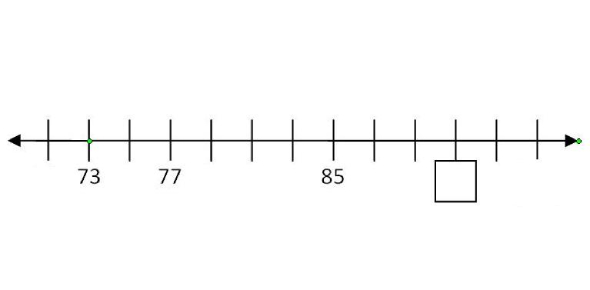# TABE Application Level M : Trivia Quiz

50 Questions | Total Attempts: 624SettingsCreate your own Quiz.

• 1.
Luis has 19 soft drink cans. His coolers each have space for 6 cans. How many coolers can he fill completely?
• A.

3

• B.

4

• C.

5

• D.

6

• 2.
Which of these numbers is a commom factor of 18 and 24?
• A.

3

• B.

4

• C.

5

• D.

7

• 3.
Which number is a multiple of 7?
• A.

17

• B.

28

• C.

37

• D.

45

• 4.
Which number goes in the blanks to make both numbers sentences true? 11 - __ = 7 7 + __ =11
• A.

4

• B.

5

• C.

6

• D.

7

• 5.
Lee has finished reading 4 out of 10 lessons from his assignment. Which fractional part of the lessons has he completed?
• A.

1/10

• B.

1/5

• C.

2/3

• D.

2/5

• 6.
Which number goes in the box on the number line?
• A.

89

• B.

91

• C.

93

• D.

87

• 7.
Marty needs to pack 32 glasses. Each box holds 6 glasses. How many boxes will be completely full?
• A.

4

• B.

5

• C.

6

• D.

7

• 8.
The cost of carrots per pound is
• A.

59 dollars

• B.

72 dollars

• C.

59 cents

• D.

98 cents

• 9.
The cost of tomatoes per pound is
• A.

2 cents

• B.

7 cents

• C.

59 cents

• D.

72 cents

• 10.
Lynn had 12 stamps, but she used 3 of them to mail letters. Which expression shows how many stamps she still has?
• A.

3 × 12

• B.

12 ÷ 3

• C.

12 - 3

• D.

3 + 12

• 11.
LaTina is saving \$60 a month for a vacation. She needs to save \$540 dollars. How long will it take her to save enough for her vacation?
• A.

6 months

• B.

9 months

• C.

12 months

• D.

18 months

• 12.
Anna saves \$75 every month. How much will she save in 16 months?
• A.

\$91

• B.

\$450

• C.

\$900

• D.

\$1,200

• 13.
Ron wrote a check \$37.80. Before he wrote the check, his balance was \$137.75. What is his new balance?
• A.

100.15

• B.

100.00

• C.

99.95

• D.

175.55

• 14.
Ella spends \$50 a month for her cell phone. How much does she pay for her cell phone in a year?
• A.

\$500

• B.

\$600

• C.

\$1,000

• D.

\$1,200

• 15.
Which of these decimals when rounded to the nearest whole number is 54?
• A.

54.81

• B.

52.65

• C.

53.85

• D.

53.40

• 16.
A dozen pens costs \$5.99. About how much does one pen cost?
• A.

\$0.50

• B.

\$0.40

• C.

\$1.00

• D.

\$1.50

• 17.
Which of these is the best estimate for the width of a piece of paper?
• A.

1 centimeter

• B.

25 centimeters

• C.

1 meter

• D.

5 meters

• 18.
Jason's average hits per game for the last three months are: 3.6, 4.5, and 6.2. What are his averages, rounded to the nearest whole number?
• A.

3, 4 and 6

• B.

4, 4 amd 6

• C.

4, 5, and 6

• D.

4, 5, and 7

• 19.
Anya spent \$32 of the \$63 she received for her birthday. She spent about
• A.

1/4 of the amount

• B.

1/3 of the amount

• C.

1/2 of the amount

• D.

2/3 of the amount

• 20.
Which of these measurements is about the same as 100 inches? (Hint: 1 in = 2.54 cm)
• A.

40 centimeters

• B.

250 centimeters

• C.

5 meters

• D.

30 meters

• 21.
What temperature does the thermometer show?
• A.

24 degrees

• B.

26 degrees

• C.

28 degrees

• D.

29 degrees

• 22.
What temperature does the thermometer show?
• A.

82 degrees

• B.

84 degrees

• C.

88 degrees

• D.

89 degrees

• 23.
Which of these measures is closest to length of your thumb?
• A.

1 centimeter

• B.

1 inch

• C.

7 centimeters

• D.

20 inches

• 24.
An airplane is scheduled to leave one city at 10:35 a.m. and arrive at another city at 12:15p.m. How long is the scheduled flight?
• A.

1 hour and 20 minutes

• B.

1 hour and 40 minutes

• C.

2 hours and 20 minutes

• D.

2 hours and 45 minutes

• 25.
Rick decided to run in a marathon, which is slighty more than 26 miles long. About how many kilometers will he run? (Hint: 1 mi = 1.6 km)
• A.

4 kilometers

• B.

10 kilometers

• C.

41 kilometers

• D.

150 kilometers

Related TopicsBack to top
×

Wait!
Here's an interesting quiz for you.